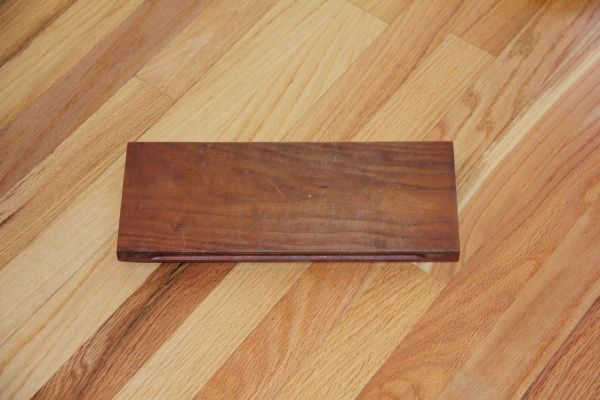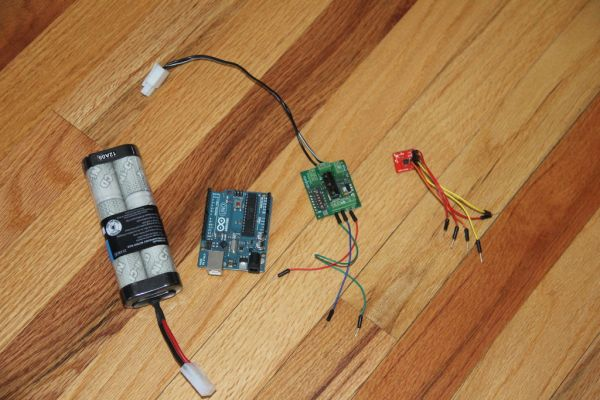# Self Balancing Segway Instructabot

### Major Components in Project

Supplies:

1.  Arduino:  I used an Uno
2.  7.2v battery
3.  Set of motors:  Parralax’s 7.2v motor set  http://www.robotshop.com/parallax-7-2v-motor-bracket-wheel-kit-pair.html
5.  Sabertooth 5X2 motor controller   http://www.robotshop.com/productinfo.aspx?pc=RB-Dim-19&lang=en-US
6.  base board
7.  3/4″ threaded rod and nuts for balance
8.  Zip ties
9.  Screws

Tools:

1.  Drill
2.  3/4″ and 1/4″ drill bits

## Step 1: Instructabot PlatformI used a piece of wood 6″ x 12″ x 0.5

## Step 2: ElectronicsNow for the electronics…

The brain of the robot is an Arduino UNO which reads the accelerometer and gives commands to the motor controller which in turn powers the motors.  The Arduino takes 10 readings per second from the accelerometer and proportionally changes the motor speed and direction.  The Arduino code has gone through many changes and tests, but you still may have to change some of the values to be compatible with your robot.  To run the robot, hook up all the wires in the correct fashion and upload the code below to the Arduino.  It should start working right there and then.

//Self Balancing Instructabot 2013
//Ferris

#include
int x, r, l, s, f, xa, xb, xc;

//Digital pin 13 is serial transmit pin to sabertooth
#define SABER_TX_PIN  13
//Not used but still initialised, Digital pin 12 is serial receive from Sabertooth
#define SABER_RX_PIN  12
//set baudrate to match sabertooth dip settings
#define SABER_BAUDRATE  9600
SoftwareSerial SaberSerial = SoftwareSerial (SABER_RX_PIN, SABER_TX_PIN );

void initSabertooth (void)  {
//communicate with sabertooth
pinMode ( SABER_TX_PIN, OUTPUT );
SaberSerial.begin( SABER_BAUDRATE );
}

void setup()                    // run once, when the sketch starts
{
initSabertooth();
Serial.begin(9600);
}

void set_motor()   {

//smooth x by averaging 3 readings of x
xa = x;
delay (20);
xb = x;
delay (20);
xc = x;
x= (xa +xb + xc)/3;

//SABER_right_FULL_FORWARD 127
//SABER_right_FULL_REVERSE 1
//SABER_left_FULL_FORWARD 255
//SABER_left_FULL_REVERSE 128

//s=slope with less being more aggressive
s = 1.8  ;
//f=fudge factor
f = 5;

//stable x around 330
if ((x > 325) && (x < 335)) {
r = 62;
l = 194;
}
//drive forward at a steady speed if leaning forward a little 310 > x < 330
if ((x > 310) && (x < 326)) {
r = 45;
l = 167;
}
//if falling forward more increase speed linearly for 279 > x < 311
if ((x > 279) && (x < 311)) {
//higher values make it faster
r = s * x – 278 + f;
l = s * x – 148 + f;
}
//if full forward x < 280
if ((x > 250) && (x < 280)) {
r = 6;
l = 133;
}
// drive backward at a steady speed if leaning back a little 334 > x > 349
if ((x > 334) && (x < 349)) {
r = 78;
l = 208;
}
//if falling backwords more increase speed linearly for 348 < x < 390
if ((x > 348) && (x < 391)) {
//lower values make it faster
r = s * x – 270 + f;
l = s * x – 140 + f;
}
//if full backwords 390 < x
if ((x > 390) && (x < 410)) {
r = 122;
l = 250;
}

//send motor outputs to sabertooth
SaberSerial.write(byte(r));
SaberSerial.write(byte(l));

}

void loop ()  {

float level = 0;

int u;

set_motor();

} // end loop

For more detail: Self Balancing Segway Instructabot

#### This Post / Project can also be found using search terms:

• segway arduino projekt